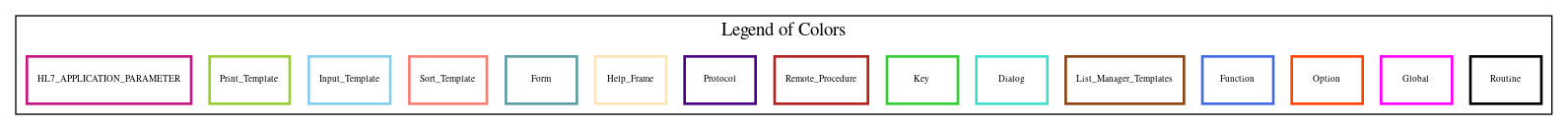Home   Package List   Routine Alphabetical List   Global Alphabetical List   FileMan Files List   FileMan Sub-Files List   Package Component Lists   Package-Namespace Mapping
Routine: PSJXR518

# Routine: PSJXR518

## Information

PSJXR518 ; COMPILED XREF FOR FILE #55.07 ; 10/28/97

## Source Information

Source file <PSJXR518.m>

## Call Graph### Call Graph Total: 4

Package Total Call Graph
Inpatient Medications 3 KILL^PSGAL5  EN^PSGAMSA  ^PSJXR519
VA FileMan 1 ^DICR

## Caller Graph

### Legends:#### Package Component Superscript legend

 action A extended action Ea event driver Ed subscriber Su protocol O limited protocol LP run routine RR broker B edit E server Se print P screenman SM inquire I### Caller Graph Total: 2

Package Total Caller Graph
Inpatient Medications 2 PSJXR5  PSJXR517

A1 ;
A
1 ;
B
2 ;
END

## External References

Name Field # of Occurrence
^DICR 2+17, 2+27, 2+33
KILL^PSGAL5 2+3, 2+7, 2+9, 2+11, 2+13, 2+21, 2+29, 2+37
EN^PSGAMSA 2+19, 2+23, 2+35
^PSJXR519 END

## Global Variables Directly Accessed

Name Line Occurrences  (* Changed,  ! Killed)
^DD(55.07 2+17, 2+27, 2+33
^PS(55 - [#55] AB2+1, 2+5*, 2+17, 2+27, 2+33

## Label References

Name Line Occurrences
2 A1+1
@( A1+1
A B
B 2+38
END A

## Naked Globals

Name Field # of Occurrence
^(0 2+17*, 2+27*, 2+33*

## Local Variables

### Legend:

 >> Not killed explicitly * Changed ! Killed ~ Newed

Name Field # of Occurrence
>> D0 2+17*, 2+27*, 2+33*
>> D1 2+17*, 2+27*, 2+33*
>> D2 2+17*, 2+27*, 2+33*
>> DA PSJXR518+2*, A1+1*, B*, 2+1, 2+5, 2+17, 2+19, 2+23, 2+27, 2+33
2+35
>> DA(1 PSJXR518+2*, A1+1*, A*, B2+1, 2+5, 2+17, 2+19, 2+23, 2+27
2+33, 2+35
>> DA(2 A1+1*, AB2+1, 2+5, 2+17, 2+19, 2+23, 2+27, 2+33
2+35
>> DIG 2+17*, 2+27*, 2+33*
>> DIH 2+17*, 2+27*, 2+33*
DIK 2+16~, 2+26~, 2+32~
DIKLM A1+1!*, 2+38
>> DIKM1 A1+1, B
>> DIKZ(0 2+1*, 2+2, 2+4, 2+6, 2+8, 2+10, 2+12, 2+14, 2+18, 2+20
2+22, 2+24, 2+28, 2+30, 2+34, 2+36
DIN 2+16~, 2+26~, 2+32~
>> DISET A1+1, 2+38
DIU 2+16~, 2+17*, 2+26~, 2+27*, 2+32~, 2+33*
DIU(0 2+3, 2+7, 2+9, 2+11, 2+13, 2+19, 2+21, 2+23, 2+29, 2+35
2+37
DIV 2+16~, 2+17!*, 2+26~, 2+27!*, 2+32~, 2+33!*
DIV(0 2+17*, 2+33*
DIV(1 2+17*, 2+27*, 2+33*
DIV(2 2+17*, 2+27*, 2+33*
PSGAL 2+3!, 2+7!, 2+9!, 2+11!, 2+13!, 2+21!, 2+29!, 2+37!
PSGAL(701 2+3
PSGAL(702 2+7
PSGAL(705 2+9
PSGAL(706 2+11
PSGAL(707 2+13
PSGAL(709 2+21
PSGAL(710 2+29
PSGAL(712 2+37
U 2+2, 2+4, 2+6, 2+8, 2+10, 2+12, 2+14, 2+18, 2+20, 2+22
2+24, 2+28, 2+30, 2+34, 2+36
>> X 2+2*, 2+3, 2+4*, 2+5, 2+6*, 2+7, 2+8*, 2+9, 2+10*, 2+11
2+12*, 2+13, 2+14*, 2+15, 2+17, 2+18*, 2+19, 2+20*, 2+21, 2+22*
2+23, 2+24*, 2+25, 2+27, 2+28*, 2+29, 2+30*, 2+31, 2+33, 2+34*
2+35, 2+36*, 2+37